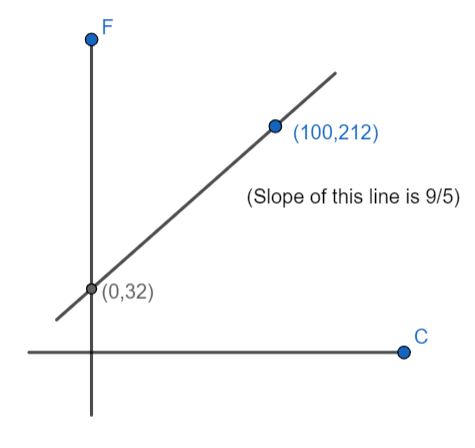QUESTION

# Convert the given temperature of ${}^\circ F$ to ${}^\circ C$.$48{}^\circ F$

Hint: To convert Fahrenheit to Celsius we will subtract 32 from given Fahrenheit temperature and multiply it by 0.556 or $\dfrac{5}{9}$. So, if we have to convert $48{}^\circ F$ to Celsius then, it is converted as. $(48-32)\times \dfrac{5}{9}$.

It is given in the question that we have to convert $48{}^\circ F$ to Celsius. So, to convert $48{}^\circ F$ into ${}^\circ C$ we will use the conversion formula. The following is the direct formula to convert Fahrenheit into Celsius.
$C=(F-32)\times \dfrac{5}{9}$ ……….(i)
Proof:
We can write $F=\dfrac{9}{5}C+x$. It is the straight line that can be represented as,
$y=(m)(C)+x$ ……….(ii)
Now, we take an example of water boiling point and freezing point to find the value of $(m)$ which is slope and $x$ is constant in equation (ii). So, water freezing point is $0{}^\circ C,32{}^\circ F$ and water boiling point is $100{}^\circ C,212{}^\circ F$.
$m=\dfrac{{{F}_{2}}-{{F}_{1}}}{{{C}_{2}}-{{C}_{1}}}$
$m=\dfrac{212-32}{100-0}=\dfrac{180}{100}=\dfrac{9}{5}$.
Thus, the slope of the line is $\dfrac{9}{5}$. Now, we can find the value of $b$, if we assume $F=32$ in equation (ii), we get
$32=\dfrac{9}{5}(0)+b$
$b=32$.
Then, we get $F=\dfrac{9}{5}(C)+32$. From this, we get
$C=(F-32)\times \dfrac{5}{9}$
$\Rightarrow 48{}^\circ F=(48-32)\times \dfrac{9}{5}$
$=16\times \dfrac{9}{5}$
$=8.89{}^\circ C$.

Note: You can convert ${}^\circ C\to {}^\circ F$ or ${}^\circ F\to {}^\circ C$ by directly using the below conversion formula.
$F=\dfrac{9}{5}(C)+32$
Because, $0{}^\circ C=32{}^\circ F$. Celsius scale is used in most of the countries and Fahrenheit scale is used in the US. We can represent Fahrenheit scale and Celsius scale as.